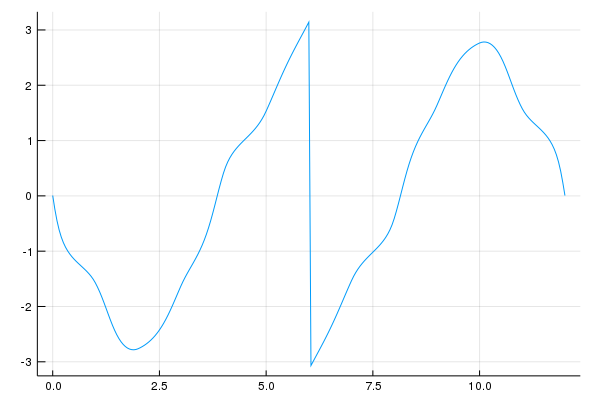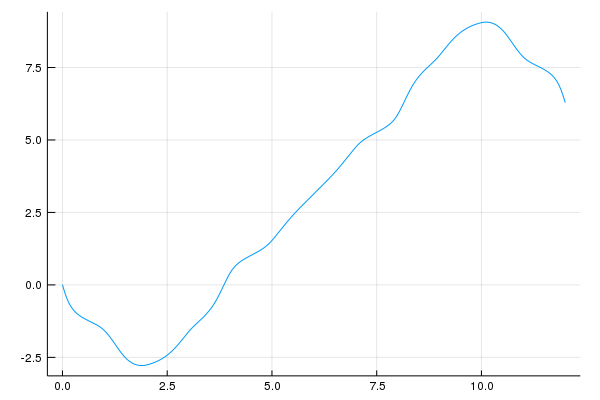0

0

1

1

# SimpleToolsMiscellaneous code that is possibly useful for my `SimpleWorld` modules.

## Notice

Several items dealing with linear algebra the were formerly in this package are moved to my `LinearAlgebraX` package.

Items include:

• `eye`
• Determinant functions
• Characteristic polynomial `char_poly`

## Complex `mod`

We have extended `mod` to that the first argument can be a Gaussian integer:

``````julia> mod(28-8im, 5)
3 + 2im
``````

## Composition of Dictionaries

Dictionaries are like functions and so it makes sense to define a composition operation for them.

Suppose that `f` and `g` are dictionaries such that all the values in `f` appear as keys in `g`. Then, if `k` is any key of `f` the expression `g[f[k]]` is defined. In this case, we may compute `g*f` to yield a new dictionary `h` with the same keys as `f` and for which `h[k] == g[f[k]]` for all keys `k`.

Warnings are issued under the following circumstances:

• If some value of `f` is not a key of `g`.
• If the type of the values in `f` doesn't match the key type for `g`.

#### Examples

Here is an example without any warnings.

``````julia> f = Dict([("alpha", 1), ("bravo", 2)])
Dict{ASCIIString,Int64} with 2 entries:
"alpha" => 1
"bravo" => 2

julia> g = Dict([(1,3.14), (2,2.718), (3,1.618)])
Dict{Int64,Float64} with 3 entries:
2 => 2.718
3 => 1.618
1 => 3.14

julia> g*f
Dict{ASCIIString,Float64} with 2 entries:
"alpha" => 3.14
"bravo" => 2.718
``````

And this is an example in which problems arise that are not so serious that the composition fails:

``````julia> f = Dict([("alpha", 1), ("bravo", 2)])
Dict{ASCIIString,Int64} with 2 entries:
"alpha" => 1
"bravo" => 2

julia> g = Dict([(1.0, 3.33)])
Dict{Float64,Float64} with 1 entry:
1.0 => 3.33

julia> g*f
WARNING: Dictionary type mismatch
WARNING: 1 keys were not mapped
Dict{ASCIIString,Float64} with 1 entry:
"alpha" => 3.33
``````

## Continuity restored

If one records the angle of a tangent vector as it traverses around a smooth closed curve, the values should be continuous. However, because there is a 2π ambiguity, one could see jumps. Here is an example.If the angles are held in an array named `y` then the following will correct the problem.

``````julia> make_continuous!(y,2pi)
``````

The resulting graph looks like this:## Flush printing

The `flush_print` function right (or left) justifies its argument in a `String` of a given number of characters.

• `flush_print(x,width)` returns a `String` version of `x` right justified in a string of length `width`.
• Use `flush_print(x,width,false)` for left-justified. ```html julia> flush_print("hello", 10) " hello" julia> flush_print("hello", 10, false) "hello " julia> flush_print(sqrt(10),30) " 3.1622776601683795" julia> flush_print(sqrt(10),5) ┌ Warning: Trunctated to fit width └ @ SimpleTools ~/.julia/dev/SimpleTools/src/flush_print.jl:9 "3.162" ```

## Print matrices for inclusion in Sage

The `sage` function takes a one or two-dimensional matrix and outputs it in a way that can be copied and pasted into a Sage session.

``````julia> v = collect(1:5)
5-element Array{Int64,1}:
1
2
3
4
5

julia> sage(v)
Matrix([,,,,])
``````
``````sage: Matrix([,,,,])
....:





sage:
``````

## Block diagonal concatenation of matrices

For matrices `A` and `B` the function `dcat(A,B)` returns a new matrix of the form `[A 0; 0 B]` where the two `0`s are zero blocks of the appropriate size. The function `dcat` can be called with any positive number of arguments.

``````julia> A = ones(Int,2,3)
2×3 Array{Int64,2}:
1  1  1
1  1  1

julia> dcat(A,2A)
4×6 Array{Int64,2}:
1  1  1  0  0  0
1  1  1  0  0  0
0  0  0  2  2  2
0  0  0  2  2  2

julia> dcat(A,2A')
5×5 Array{Int64,2}:
1  1  1  0  0
1  1  1  0  0
0  0  0  2  2
0  0  0  2  2
0  0  0  2  2

julia> dcat(A,2A,3A)
6×9 Array{Int64,2}:
1  1  1  0  0  0  0  0  0
1  1  1  0  0  0  0  0  0
0  0  0  2  2  2  0  0  0
0  0  0  2  2  2  0  0  0
0  0  0  0  0  0  3  3  3
0  0  0  0  0  0  3  3  3
``````

06/27/2016